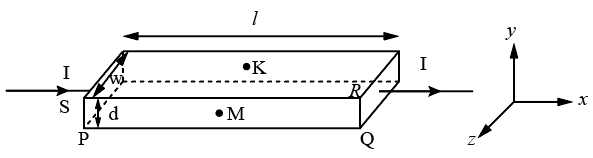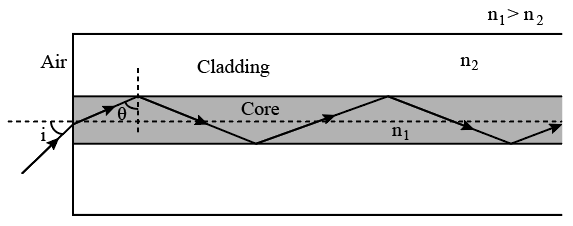Instructions

For the following questions answer them individually

Question 11

Question 12

Question 13

Question 14

Question 15

Question 16

# A fission reaction is given by $$_{92}^{236}U \rightarrow _{54}^{140}Xe + _{38}^{94}Sr + x + y$$ where x and y are two particles. Considering $$_{92}^{236}U$$ to be at rest, the kinetic energies of the products are denoted by $$K_{Xe}, K_{Sr}, K_x (2 MeV)$$ and $$K_y (2 MeV),$$ respectively. Let the binding energies per nucleon of $$_{92}^{236}U, _{54}^{140}Xe$$ and $$_{38}^{94}Sr$$ be 7.5 MeV, 8.5 MeV and 8.5 MeV,respectively. Considering different conservation laws, the correct option(s) is(are)

Instructions

In a thin rectangular metallic strip a constant current I flows along the positive x-direction, as shown in the figure. The length, width and thickness of the strip are l, w and d, respectively.
A uniform magnetic field $$\overrightarrow{B}$$ is applied on the strip along the positive y-direction. Due to this, the charge carriers experience a net deflection along the z-direction. This results in accumulation of charge carriers on the surface PQRS and appearance of equal and opposite charges on the face opposite to PQRS. A potential difference along the z-direction is thus developed. Charge accumulation continues until the magnetic force is balanced by the electric force. The current is assumed to be uniformly distributed on the cross section of the strip and carried by electrons.Question 17

Question 18

# Consider two different metallic strips (1 and 2) of same dimensions (length l, width w and thickness d) with carrier densities $$n_1$$ and $$n_2$$, respectively. Strip 1 is placed in magnetic field $$B_1$$ and strip 2 is placed in magnetic field $$B_2,$$ both along positive y-directions. Then $$V_1$$ and $$V_2$$ are the potential differences developed between K and M in strips 1 and 2, respectively. Assuming that the current I is the same for both the strips, the correct option(s) is(are)

Instructions

Light guidance in an optical fiber can be understood by considering a structure comprising of thin solid glass cylinder of refractive index $$n_1$$ surrounded by a medium of lower refractive index $$n_2$$. The light guidance in the structure takes place due to successive total internal reflections at the
interface of the media $$n_1$$ and $$n_2$$ as shown in the figure. All rays with the angle of incidence i less than a particular value $$i_m$$ are confined in the medium of refractive index $$n_1$$. The numerical aperture (NA) of the structureis defined as sin $$i_m$$.Question 19

Question 20

OR